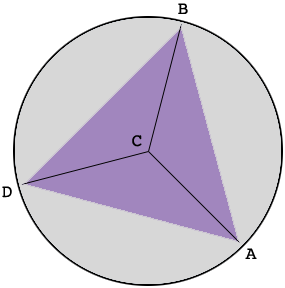SEARCH HOMEMath Central Quandaries & QueriesQuestion from Penny: What is the length of each side of the largest equilateral triangle that fits inside a 3 inch diameter circle?Hi Penny,

In my diagram $C$ is the center of the circle and the vertices of the equilateral triangle are $A, B$ and $C.$What do you know about triangle $ABC?$

PennyMath Central is supported by the University of Regina and The Pacific Institute for the Mathematical Sciences.